xTras Tutorial Functions »|Tutorials »

# The Gauss-Bonnet term

In this tutorial we will check that the Euler density in 4 dimensions, also known as the Gauss-Bonnet term, is topological. That is, we will show that its equations of motion are zero.
 EulerDensity[cd] gives the Euler density associated to the covariant derivative cd. VarL[g[-a,-b]][L] returnswhile integrating by parts with respect to the covariant derivative of the metric gab. FullSimplification[][expr] tries to simplify expr as much as possible, taking Bianchi identities into account and sorting covariant derivatives. ConstructDDIs[expr] constructs all scalar dimensional dependent identities that can be build out of expr. SolveTensors[expr] attempts to solve the system expr of tensorial equations for all tensors in expr. MakeAnsatz[{e1,e2,...}] returns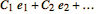, where the's are newly defined constant symbols. SolveConstants[expr] attempts to solve the system expr of tensorial equations for all constant symbols appearing in expr.

Functions used in this tutorial.

First, load the package and define the manifold and metric.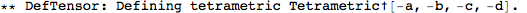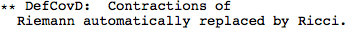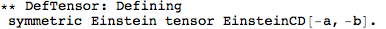The Gauss-Bonnet term can be obtained from the function EulerDensity:
 Out=Note that EulerDensity omits the square root of the metric determinant, so technically GBterm isn't a density.
Next, we determine the equations of motion of the Gauss-Bonnet term with VarL:
 Out=Because the Gauss-Bonnet term is topogical, the above should identically be zero. There are no further simplifications coming from Bianchi identities that we can use: FullSimplification took care of most of them, and if there were some remaining we still couldn't use them to get rid of the Ricci tensors.
So the above equations of motion can only be zero due to dimensionally dependent identities. We can obtain the relevant identities with a call to ConstructDDIs:Out=This constructed all dimensionally dependent identities that have two Riemann tensors (or contractions thereof) and free indices a and b. All of these four expression are zero. Even though there are four identities, only two of them are independent (not taking multi-term symmetries into account). This can be verified with SolveTensors: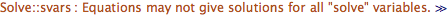Out=The two options are needed to prevent SolveTensors from making rules for every index combination on the left-hand-side related by symmetries (UseSymmetries) and by raising and lowering of the indices (MetricOn).
The above output consists of rules that we can use to enforce the identities on the equations of motion:
 Out=So indeed the equations of motion are zero.
Alternatively, we could have made an Ansatz with arbitrary coefficients from the identities with MakeAnsatz,
 Out=and tried to make this equal to the equations of motion by solving for the coefficients with SolveConstants:Out=So again, the equations of motion are equal to particular linear combination of the dimensionally dependent identities, and hence they are zero.